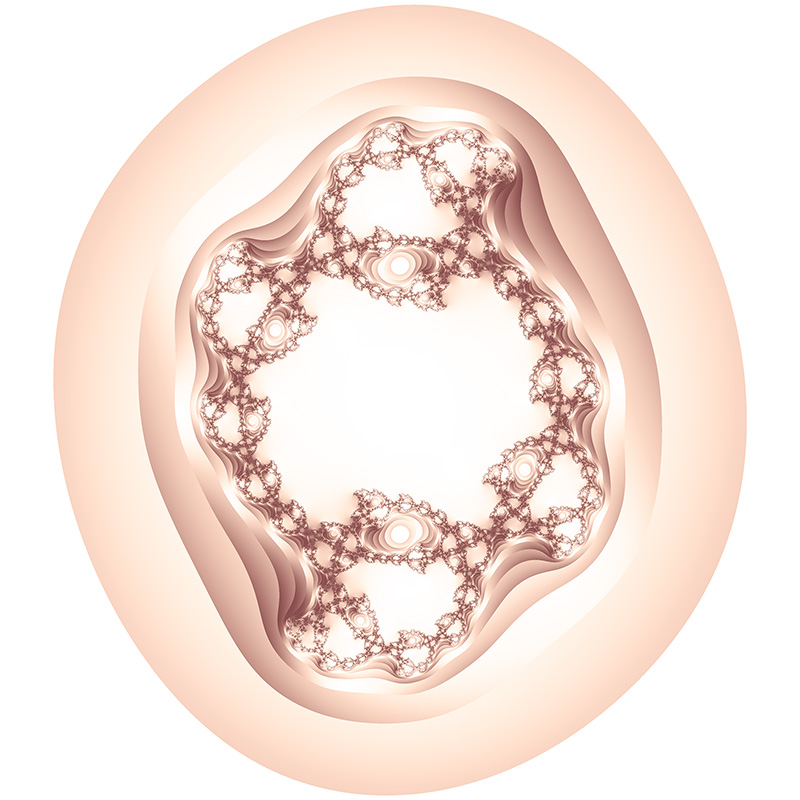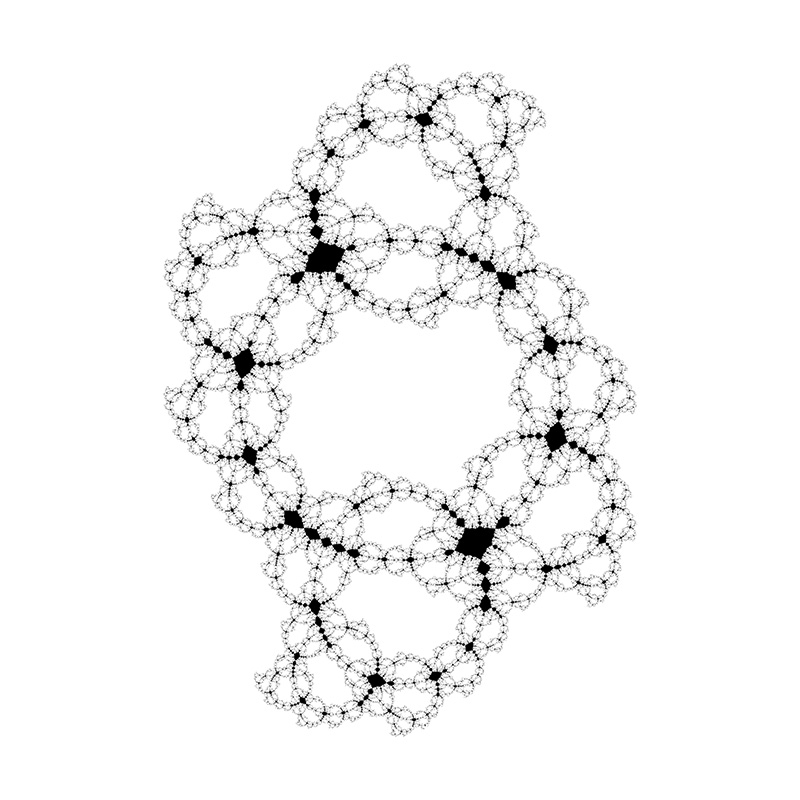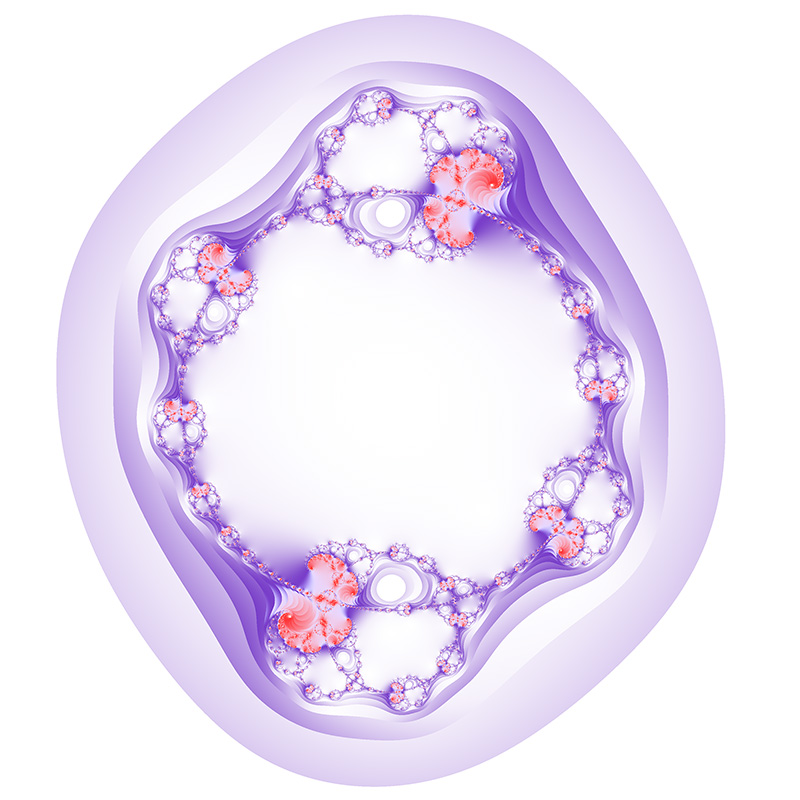# Rings fractal

Written by Paul Bourke
Inspired by Roger Bagula
July 2019

The following are created by considering the behavior of the following series, where z0 is the point in the complex plane being imaged.Sigma = sqrt(2)Sigma = sqrt(3) and plotting grey level by the time for the series to escape to infinity, like a standard Mandelbrot.In reality sigma can be "anything", the following is sqrt(1.5).For most of the images the straight escape time is not used but rather the magnitude of the complex vector sum of the series. That is, each term of the series is added vector wise and the colour assigned depending on the magnitude. In the following the escape time image (dark portions) modulates the vector sum (red portions).